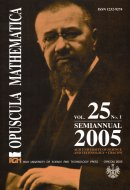Opuscula Math. 25, no. 1 (2005), 29-99

Opuscula Mathematica

# Monotone iterative methods for infinite systems of reaction-diffusion-convection equations with functional dependence

Stanisław Brzychczy

Abstract. We consider the Fourier first initial-boundary value problem for an infinite system of semilinear parabolic differential-functional equations of reaction-diffusion-convection type of the form $\mathcal{F}^i[z^i](t,x)=f^i(t,x,z),\quad i \in S,$ where $\mathcal{F}^i:=\mathcal{D}_t-\mathcal{L}^i,\quad \mathcal{L}^i:=\sum_{j,k=1}^m a_{jk}^i(t,x)\mathcal{D}^2_{x_jx_k}+\sum_{j=1}^m b_j^i(t,x)\mathcal{D}_{x_j}$ in a bounded cylindrical domain $$(0,T] \times G:=D \subset \mathbb{R}^{m+1}$$. The right-hand sides of the system are Volterra type functionals of the unknown function $$z$$. In the paper, we give methods of the construction of the monotone iterative sequences converging to the unique classical solution of the problem considered in partially ordered Banach spaces with various convergence rates of iterations. We also give remarks on monotone iterative methods in connection with numerical methods, remarks on methods for the construction of lower and upper solutions and remarks concerning the possibility of extending these methods to more general parabolic equations. All monotone iterative methods are based on differential inequalities and, in this paper, we use the theorem on weak partial differential-functional inequalities for infinite systems of parabolic equations, the comparison theorem and the maximum principle. A part of the paper is based on the results of our previous papers. These results generalize the results obtained by several authors in numerous papers for finite systems of semilinear parabolic differential equations to encompass the case of infinite systems of semilinear parabolic differential-functional equations. The monotone iterative schemes can be used for the computation of numerical solutions.

Keywords: infinite systems, reaction-diffusion-convection equations, semilinear parabolic differential-functional equations, Volterra functionals, monotone iterative methods, method of upper and lower solutions.

Mathematics Subject Classification: 35R15, 35K55, 35K57, 35R10.

Full text (pdf)

• Stanisław Brzychczy
• AGH University of Science and Technology, Faculty of Applied Mathematics, Department of Differential Equations, al. Mickiewicza 30, 30-059 Cracow, Poland